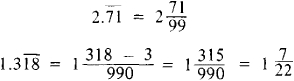# Infinite Periodic Decimal

The following article is from The Great Soviet Encyclopedia (1979). It might be outdated or ideologically biased.

## Infinite Periodic Decimal

an infinite decimal that, beginning at a certain point, consists of a periodically repeating fixed block of digits called its repeating part. An example of an infinite periodic decimal is 1.3181818 …. It is expressed in abbreviated form by placing a bar over the repeating part: 1.318. In Russian, the abbreviated form is written by placing the repeating part in parentheses: 1.3(18). An infinite periodic decimal is said to be pure if the repeating part begins immediately after the decimal point—for example, 2.71 = 2.7171 …—and mixed otherwise—for example, 1.318.

Infinite periodic decimals are important in arithmetic because the decimal representation of a rational number—that is, a fraction—is always either a terminating decimal or an infinite periodic decimal. More precisely, a terminating decimal is obtained whenever the denominator of a reduced fraction has no prime factors other than 2 and 5; if other prime factors occur, an infinite periodic decimal is obtained. The infinite periodic decimal is pure if the denominator of the fraction does not contain 2 or 5 as factors, and is mixed otherwise.

Every infinite periodic decimal is equal to some rational number and can be transformed into a fraction. A pure infinite periodic decimal is equal to a fraction whose numerator is the repeating part and whose denominator is given by the digit 9 written as many times as there are digits in the repeating part. When a mixed infinite periodic decimal is transformed into a fraction, the numerator is the difference between the numbers preceding the second and first occurrences of the repeating part, and the denominator is given by the digit 9 written as many times as there are digits in the repeating part and followed by as many zeros as there are digits before the first occurrence of the repeating part. These rules assume that the infinite periodic decimal contains no digits before the decimal point. If there are such digits, they are considered separately. For example,Rules are also known for determining the length of the repeating part of the infinite periodic decimal that corresponds to a given fraction. For example, for a fraction a/ρ, where ρ is a prime and 1 ≤ aρ – 1, the length of the repeating part is a divisor of ρ – 1. Thus, the well-known approximations 22/7 and 355/113 to the number π have repeating parts of length 6 and 112, respectively.